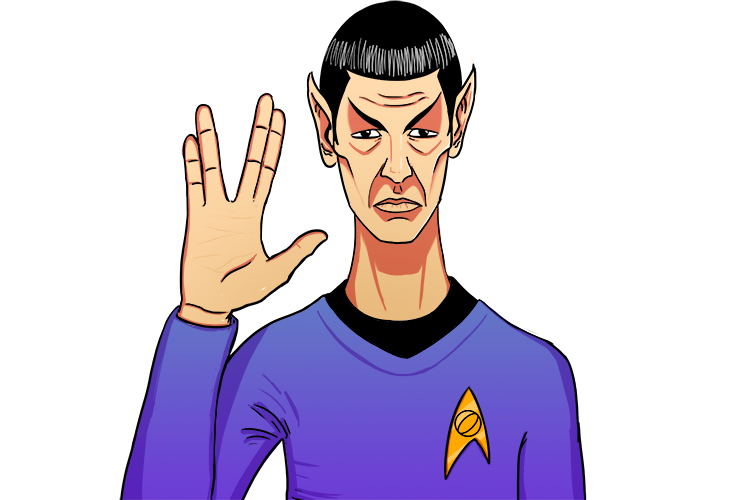# Upper and lower boundary and logic

In the following skills of upper and lower boundaries which include addition, subtraction, multiplication and division we state that you should use logic.It's just logic.

But what we mean by that is

 Addition Big number + Big number = Biggest number Small number + Small number = Smallest number Subtraction Big number - Small number = Biggest number Small number - Big number = Smallest number Multiplication Big number xx Big number = Biggest number Small number xx Small number = Smallest number Division Big number div Small number = Biggest number Small number div Big number = Smallest number

It's obvious. It's logic. Ask yourself what else could it be?